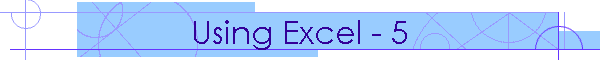Home ] What's new! ] Suspension types ] Tutorials ] Downloads ] Data files ] FAQ ] Purchase here ] Contact us ]

Reading input data from Excel, doing the calculations, and exporting the calculated data back to Excel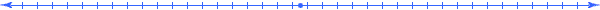This Excel tutorial uses Excel V16. Check the supported versions of Excel here.

This tutorial uses the SRD912 demo file, but you can use your own data.

This tutorial requires an Excel workbook with worksheets for both input data and calculated data.
Create worksheets named "Front input", "Rear input", "Front calc" and "Rear calc".

If you have asymmetric data then you will need to either create individual LH and RH worksheets or append the range names with "LH" or "RH".

If you are including any of the ride height input data and doing a complete vehicle calculation using VCalc then you only need to include the ride height data in one worksheet.
But if you are only calculating one end (by using any of the Calc or ECalc) then you need to include the  ride height data in the specific car end worksheet.
If you have individual LH and RH worksheets then you only need to include the ride height data in the one worksheet.

For this tutorial I have used the four basic worksheets, "Front input", "Rear input", "Front calc" and "Rear calc".

To keep it simple I will add the ride height to "Front Input" and the wheel alignment camber LH and RH to both "Front input" and "Rear input".
For the calculated data, I will add the wheel alignment camber in bump and droop for both "Front calc" and "Rear calc" LH and RH.

This is the range data.

 S3D_Vehicle_RideHeight 'Front input'!\$B\$2:\$B\$5 S3D_WheelAlignment_Item1_LH 'Front input'!\$B\$8 S3D_WheelAlignment_Item1_LH 'Front input'!\$B\$9 S3D_WheelAlignment_Item1_LH 'Rear input'!\$B\$8 S3D_WheelAlignment_Item1_RH 'Rear input'!\$B\$9 S3D_B_Point_Bump 'Front calc'!\$A\$2:\$A\$16 S3D_B_Point_Camber_LH 'Front calc'!\$B\$2:\$B\$16 S3D_B_Point_Camber_RH 'Front calc'!\$C\$2:\$C\$16 S3D_B_Point_Bump 'Rear calc'!\$A\$2:\$A\$16 S3D_B_Point_Camber_LH 'Rear calc'!\$B\$2:\$B\$16 S3D_B_Point_Camber_RH 'Rear calc'!\$C\$2:\$C\$16

Start SusProg3D, and open the demo file, SRD912.s3d
SusProg3D is set to [Front] and [LH]

Go to the Tools tab, and then both Calc2Excel and Excel2Input. This will open the "Export calculated data to Excel" and the "Import input data from Excel" dialogs, and connect to the open workbook.

Update the worksheet reference inputs to the correct worksheets.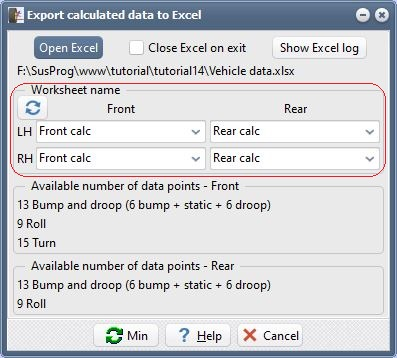Open an ECalc and tick Roll and Bump. You can include any other items, but for this tutorial we only need the Roll and Bump calc.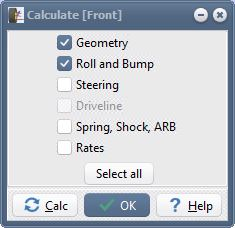Using ECalc, do a Calc.
ECalc will then call Calc2Excel to update the workbook, and update the Travel and Camber cells. Verify that the camber data has been written to the 'Front calc' worksheet, and that the 'Front input', 'Rear input' and 'Rear calc' worksheets are still blank.
This is to verify that Calc2Excel is working correctly.

Go to the Tools tab, and Excel2Input. This will open the "Import input data from Excel" dialog, and connect to the open workbook.

Update the worksheet reference inputs to the correct worksheets.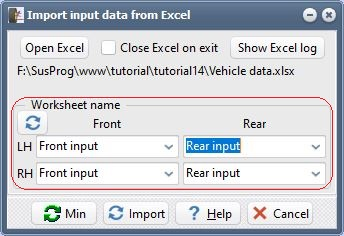Back to Excel.

Clear all the data on the 'Front calc' worksheet.
In the 'Front input' worksheet add 0.00 for the LH Camber, -1.00 for the RH Camber, 140.0 for the front ride heights and 165.0 for the rear ride heights.
Note that if you have defined input name ranges, and they are left blank in Excel, then they will be imported into SusProg3D as zero.

Now in the "Import input data from Excel" dialog, click on Import.
This will update the wheel alignment and ride height and then call ECalc to do the calculations. ECalc will then call Calc2Excel and export the calculated data back to Excel.

Now we will use the VCalc dialog. The difference is that ECalc does only one end of the vehicle whereas VCalc does both ends of the vehicle.
Close ECalc and open VCalc and tick Roll and Bump. You can include any other items, but for this tutorial we only need the Roll and Bump calc. Then both front and rear worksheets will be updated.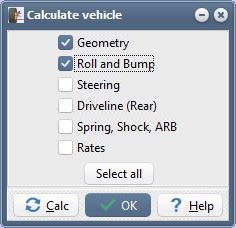Back to Excel.

Clear all the data on both the 'Front calc' and 'Rear calc' worksheets.
In the 'Front input' worksheet change the the LH Camber to 1.0, the RH Camber to 1.5, the front ride heights to 150.0 and the rear ride heights to 160.0.
In the 'Rear input' worksheet add -1.00 for LH Camber and -2.00 for RH Camber.
If you want to have other dialogs open, such as Alignment, then open them.

Now in the "Import input data from Excel" dialog, click on Import.
This will update the wheel alignment and ride height and then call VCalc to do the calculations. VCalc will then call Calc2Excel and export the calculated data back to Excel.

This tutorial has shown how we can use a single Excel workbook containing both input and calculated data, and use SusProg3D to do the calculations when the input data is changed.

Tutorial developed using Excel V16 and SusProg3D V5.106A on Windows 10 (64-bit) Nov 2020